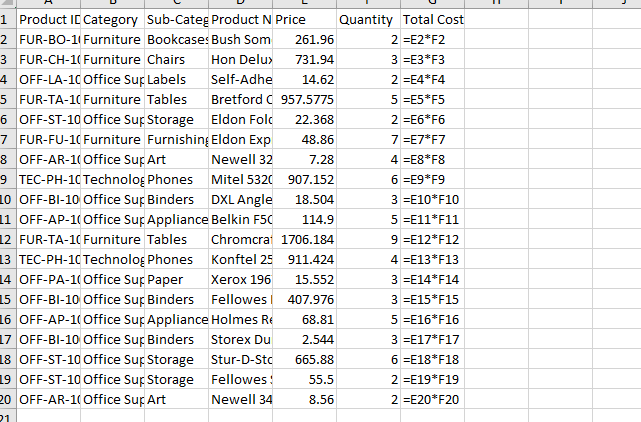# Write Range to SpreadSheet displays the formula not the result

Is it possible to calculate mathematical result inside a datatable then write it in a workbook? I have an excel table with 3 columns Price, Quantity and Total Cost. Total Cost Column is empty and needs to be calculated. I stored the entire excel table in a DataTable, then applied For Each Row on the data table and calculated the row index number for each current row which is an int32 value. I was able to write the correct formula for Total Cost Column in the data table by using rowindex value and string manipulation.

When I am writing the result in a spread sheet it displays the formula not the result. E.g. (=A2*B2). My question is how can we get the exact result not the formula?

I Was able to obtain result with Write Cell Activity, but I want to avoid it.

Uipath Project.zip (498.2 KB)Use excel application scope and try to use write range inside.

Regards,
Arivu

3 Likes

Thank you so much! It worked. Do you know why it works in Excel Application scope and did not work in Workbook?

This topic was automatically closed 3 days after the last reply. New replies are no longer allowed.